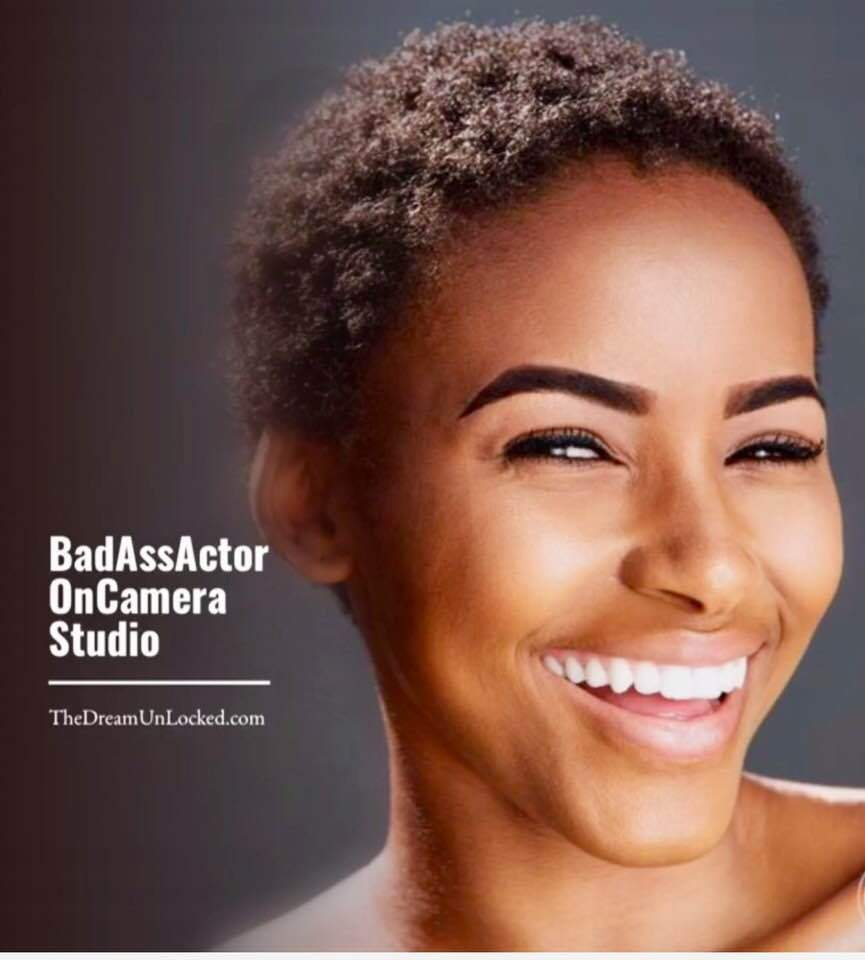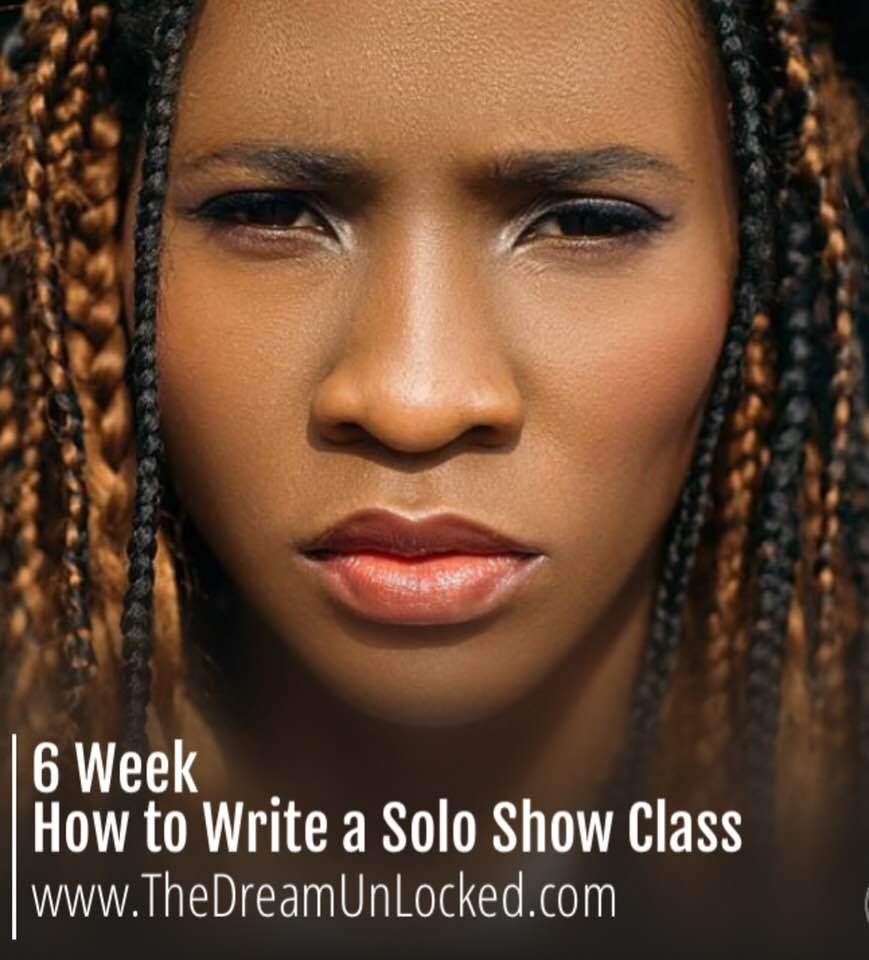table div table+table+table div table{width:100%;padding:0}table div table+table+table div table img{width:96.23%;padding:0;float:none}table div table+table+table div table td{width:100%;padding:0 1.88% 18px}/* styles */table div table+table+table+table div table{width:100%;padding:0}table div table+table+table+table div table img{width:96.23%;padding:0;float:none}table div table+table+table+table div table td{width:100%;padding:0 1.88% 18px}/* styles */Tony-winning producer, writer, actor, coach & CEO of TheDreamUnLocked April Yvette Thompson

How effective are self submissions as opposed to going in the room? I get a lot from my agent and I'm wondering if I should request receiving less of those...

This is a great question and one that Hilary Widds has been addressing a lot in the 6 Week How to Be a Working TV Actor class....i'm sure you'll get more info in the 12 Step MFA, but here's the skinny....

If you have the opportunity to go in person to meet a director or CD you've never met, GO! YOU NEED TO BUILD RELATIONSHIPS TO GET MORE AUDITIONS...once you have more credits and solid relationships, tapes are fine.

Now, these days to save money, some projects only ask for self-tapes...that too is fine...here's the thing with a self-tape, it's gotta be awesome...i mean awesome...you get one shot to wow so all the technical details need to be nailed...no questions asked...

get a rehearsal partner and practice nine times fast, then go to someone who has stellar equipment to put you on tape OR learn how to do it yourself with the correct lighting and a high quality iphone or mac computer...and learn how to do sightlines and eyelines...it's worth take a quick and dirty class or coaching session to learn how to set this up so you can do it urself...

i book almost completely from self-tapes and my reel...only because i've been submitting really strong self-tapes to the same CD's over and over again...each great self-tape is more money in the bank and more people see it: producers, studio execs, show runners, directors, DP's....it's an excellent opportunity to get known if you do it right..

Tiffany Webb

## Tiffany Webb

Mmmmm, makes perfect sense, got it! Thank you April!

# April & TheDreamUnLocked Team

 table div table+table+table+table+table+table+table+table div table{width:100%;padding:0}table div table+table+table+table+table+table+table+table div table img{width:96.23%;padding:0;float:none}table div table+table+table+table+table+table+table+table div table td{width:100%;padding:0 1.88% 18px}/* styles */table div table+table+table+table+table+table+table+table+table div table{width:100%;padding:0}table div table+table+table+table+table+table+table+table+table div table img{width:96.23%;padding:0;float:none}table div table+table+table+table+table+table+table+table+table div table td{width:100%;padding:0 1.88% 18px}/* styles */table div table+table+table+table+table+table+table+table+table+table div table{width:100%;padding:0}table div table+table+table+table+table+table+table+table+table+table div table img{width:96.23%;padding:0;float:none}table div table+table+table+table+table+table+table+table+table+table div table td{width:100%;padding:0 1.88% 18px}/* styles */## TheDreamUnLocked Coach, Hilary Ward Gives You All the Tools You Need to Self Tape# Background

▪ Get your background together. A curtain or a sheet stretched across the wall behind you is fine. Buy a cheap one from the dollar store to use for taping. Preferably not white.
 ▪ Get your background together. A curtain or a sheet stretched across the wall behind you is fine. Buy a cheap one from the dollar store to use for taping. Preferably not white.

# Lighting

▪ Light yourself from the front with a nice diffused light. Usually facing an open window is nice. Don't ever have the light behind you or harsh direct light on your face.
▪ If you want to, you can buy a light kit on Amazon for about \$70. But most of you don't need that.
▪ If you use your phone for self tapes (which you should because it has an HD camera),
▪ BUY A LIGHT RING for your camera. Here's one for \$10.99 on Amazon. LIGHT RING FOR CAMERA
▪
 ▪ Light yourself from the front with a nice diffused light. Usually facing an open window is nice. Don't ever have the light behind you or harsh direct light on your face.
 ▪ If you want to, you can buy a light kit on Amazon for about \$70. But most of you don't need that.
 ▪ If you use your phone for self tapes (which you should because it has an HD camera),
 ▪ BUY A LIGHT RING for your camera. Here's one for \$10.99 on Amazon. LIGHT RING FOR CAMERA
 ▪

# Microphone

▪ Sound issues? Use a mic.
▪ Here's a mic that plugs right into your phone. It's \$12.99.
▪ If that mic won't plug into your phone, here's an adaptor on Amazon. It's \$10
 ▪ Sound issues? Use a mic.
 ▪ Here's a mic that plugs right into your phone. It's \$12.99.
 ▪ If that mic won't plug into your phone, here's an adaptor on Amazon. It's \$10

# Tripod

▪ If you don't have a tripod, stack your camera up on some books. If you get a tripod, again, I recommend these.
▪ And buy a clip so you can attach your phone to it. This clip is \$6.
▪
 ▪ If you don't have a tripod, stack your camera up on some books. If you get a tripod, again, I recommend these.
 ▪ And buy a clip so you can attach your phone to it. This clip is \$6.
 ▪

Store all of these things together so when you need them, you know right where they are and can easily do your tape. Make it easy for yourself to practice self-taping.

 table div table+table+table+table+table+table+table+table+table+table+table+table+table+table+table div table{width:100%;padding:0}table div table+table+table+table+table+table+table+table+table+table+table+table+table+table+table div table img{width:96.23%;padding:0;float:none}table div table+table+table+table+table+table+table+table+table+table+table+table+table+table+table div table td{width:100%;padding:0 1.88% 18px}/* styles */table div table+table+table+table+table+table+table+table+table+table+table+table+table+table+table+table div table{width:100%;padding:0}table div table+table+table+table+table+table+table+table+table+table+table+table+table+table+table+table div table img{width:96.23%;padding:0;float:none}table div table+table+table+table+table+table+table+table+table+table+table+table+table+table+table+table div table td{width:100%;padding:0 1.88% 18px}/* styles */table div table+table+table+table+table+table+table+table+table+table+table+table+table+table+table+table+table div table{width:100%;padding:0}table div table+table+table+table+table+table+table+table+table+table+table+table+table+table+table+table+table div table img{width:96.23%;padding:0;float:none}table div table+table+table+table+table+table+table+table+table+table+table+table+table+table+table+table+table div table td{width:100%;padding:0 1.88% 18px}/* styles */## Artist Spotlight: Congratulations Angela Lewis for her second season as a series regular on SnowFall

 table div table+table+table+table+table+table+table+table+table+table+table+table+table+table+table+table+table+table+table div table td,table.module-18{width:100%;padding:0}table div table+table+table+table+table+table+table+table+table+table+table+table+table+table+table+table+table+table+table div table{width:100%;float:none;margin-left:auto;margin-right:auto;padding:0}table div table+table+table+table+table+table+table+table+table+table+table+table+table+table+table+table+table+table+table div table a{border:0 none;text-decoration:none}table div table+table+table+table+table+table+table+table+table+table+table+table+table+table+table+table+table+table+table div table img{width:100%!important;border:0 none;text-decoration:none}/* styles */
 /* styles */ Catch Angela Lewis in the new season of SnowFall on FX directed by John Singleton Angela is a series regular playing the fabulous Aunt Louie
 table div table+table+table+table+table+table+table+table+table+table+table+table+table+table+table+table+table+table+table+table+table div table{width:100%;padding:0}table div table+table+table+table+table+table+table+table+table+table+table+table+table+table+table+table+table+table+table+table+table div table img{width:96.23%;padding:0;float:none}table div table+table+table+table+table+table+table+table+table+table+table+table+table+table+table+table+table+table+table+table+table div table td{width:100%;padding:0 1.88% 18px}/* styles */table div table+table+table+table+table+table+table+table+table+table+table+table+table+table+table+table+table+table+table+table+table+table div table{width:100%;padding:0}table div table+table+table+table+table+table+table+table+table+table+table+table+table+table+table+table+table+table+table+table+table+table div table img{width:96.23%;padding:0;float:none}table div table+table+table+table+table+table+table+table+table+table+table+table+table+table+table+table+table+table+table+table+table+table div table td{width:100%;padding:0 1.88% 18px}/* styles */table div table+table+table+table+table+table+table+table+table+table+table+table+table+table+table+table+table+table+table+table+table+table+table+table+table div table{width:100%;padding:0}table div table+table+table+table+table+table+table+table+table+table+table+table+table+table+table+table+table+table+table+table+table+table+table+table+table div table img{width:96.23%;padding:0;float:none}table div table+table+table+table+table+table+table+table+table+table+table+table+table+table+table+table+table+table+table+table+table+table+table+table+table div table td{width:100%;padding:0 1.88% 18px}/* styles */table div table+table+table+table+table+table+table+table+table+table+table+table+table+table+table+table+table+table+table+table+table+table+table+table+table+table div table{width:100%;padding:0}table div table+table+table+table+table+table+table+table+table+table+table+table+table+table+table+table+table+table+table+table+table+table+table+table+table+table div table img{width:96.23%;padding:0;float:none}table div table+table+table+table+table+table+table+table+table+table+table+table+table+table+table+table+table+table+table+table+table+table+table+table+table+table div table td{width:100%;padding:0 1.88% 18px}/* styles */table div table+table+table+table+table+table+table+table+table+table+table+table+table+table+table+table+table+table+table+table+table+table+table+table+table+table+table div table{width:100%;padding:0}table div table+table+table+table+table+table+table+table+table+table+table+table+table+table+table+table+table+table+table+table+table+table+table+table+table+table+table div table img{width:96.23%;padding:0;float:none}table div table+table+table+table+table+table+table+table+table+table+table+table+table+table+table+table+table+table+table+table+table+table+table+table+table+table+table div table td{width:100%;padding:0 1.88% 18px}/* styles */